# How to Calculate and Solve for Period | Motion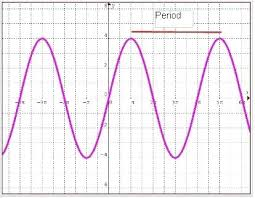The image above represents period.

To compute for period, two essential parameters are needed and these parameters are length (l) and acceleration due to gravity (g).

The formula for calculating period:

T = 2π(√(l / g))

Where;

T = Period
l = Length
g = Acceleration due to gravity

Let’s solve an example;
Find the period when the length is 4 and the acceleration due to gravity is 9.8.

This implies that;

l = Length = 4
g = Acceleration due to gravity = 9.8

T = 2π(√(l / g))
T = 2π(√(4 / 9.8))
T = 2π(√(0.408))
T = 2π(0.6388)
T = 6.28 x 0.6388
T = 4.014

Therefore, the period is 4.014 s.

Calculating the Length when the Period and the Acceleration due to Gravity is Given.

l = (T / )2 x g

Where;

l = Length
T = Period
g = Acceleration due to gravity

Let’s solve an example;
Find the length when the period is 28 and the acceleration due to gravity is 9.8.

This implies that;

T = Period = 28
g = Acceleration due to gravity = 9.8

l = (T / )2 x g
l = (28 / 6.28)2 x 9.8
l = (4.458)2 x 9.8
l = 19.87 x 9.8
l = 194.7

Therefore, the length is 194.7.

Calculating the Acceleration due to Gravity when the Period and the Length is Given.

g = l / (T / )2

Where;

g = Acceleration due to gravity
T = Period
l = Length

Let’s solve an example;
Find the acceleration due to gravity when the period is 12 and length is 3.

This implies that;

T = Period = 12
l = Length = 3

g = l / (T / )2
g = 3 / (12 / 6.28)2
g = 3 / (1.91)2
g = 3 / 3.648
g = 0.82

Therefore, the acceleration due to gravity is 0.82.

Nickzom Calculator – The Calculator Encyclopedia is capable of calculating the period.

To get the answer and workings of the period using the Nickzom Calculator – The Calculator Encyclopedia. First, you need to obtain the app.

You can get this app via any of these means:

To get access to the professional version via web, you need to register and subscribe for NGN 1,500 per annum to have utter access to all functionalities.
You can also try the demo version via https://www.nickzom.org/calculator

Apple (Paid) – https://itunes.apple.com/us/app/nickzom-calculator/id1331162702?mt=8
Once, you have obtained the calculator encyclopedia app, proceed to the Calculator Map, then click on Motion under Physics.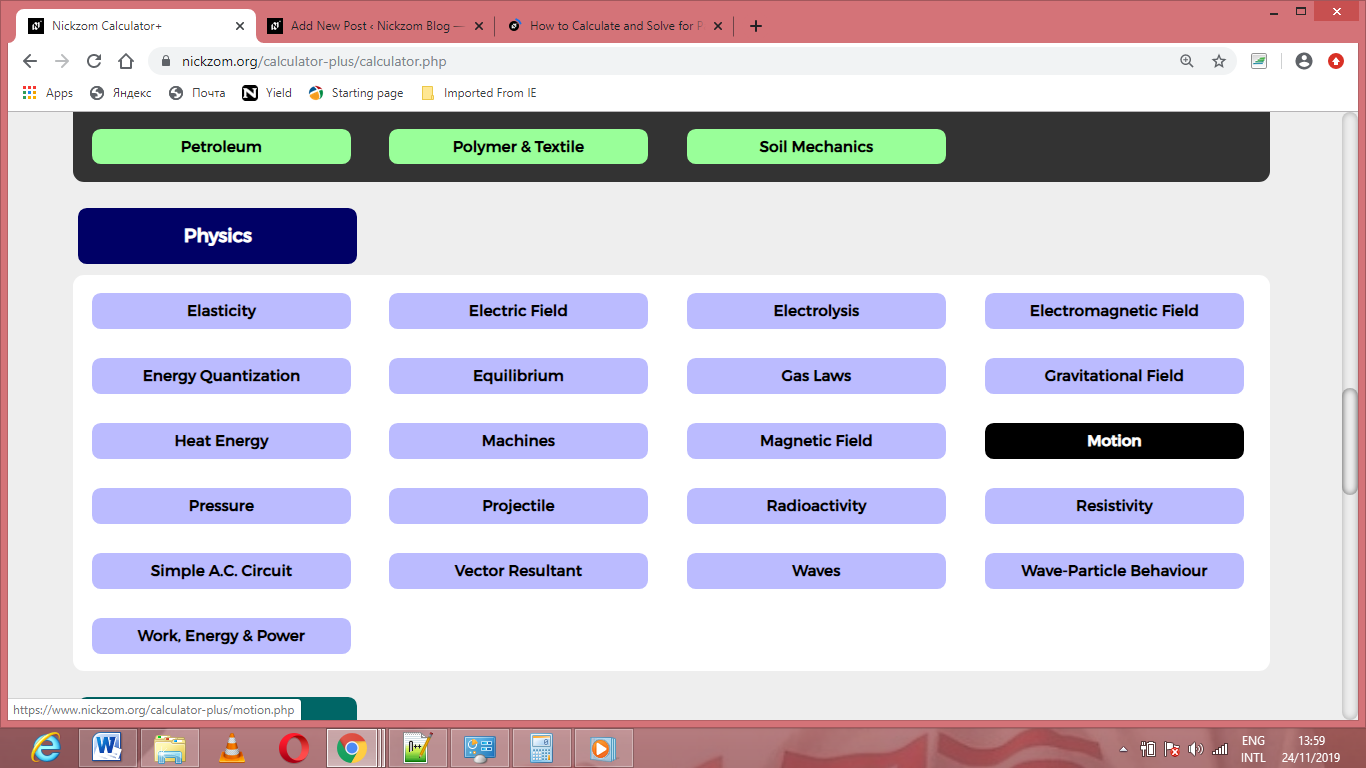Now, Click on Period under Motion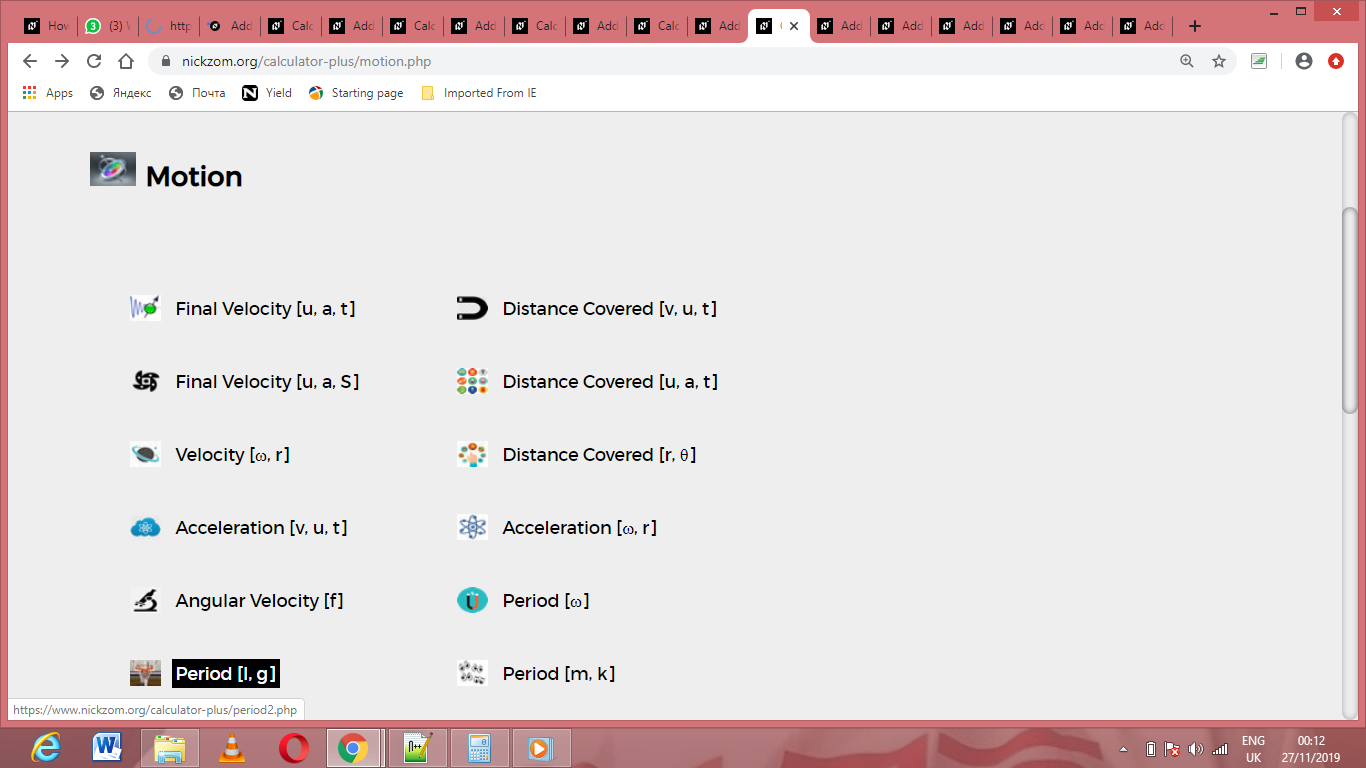The screenshot below displays the page or activity to enter your values, to get the answer for the period according to the respective parameters which are the length (l) and acceleration due to gravity (g).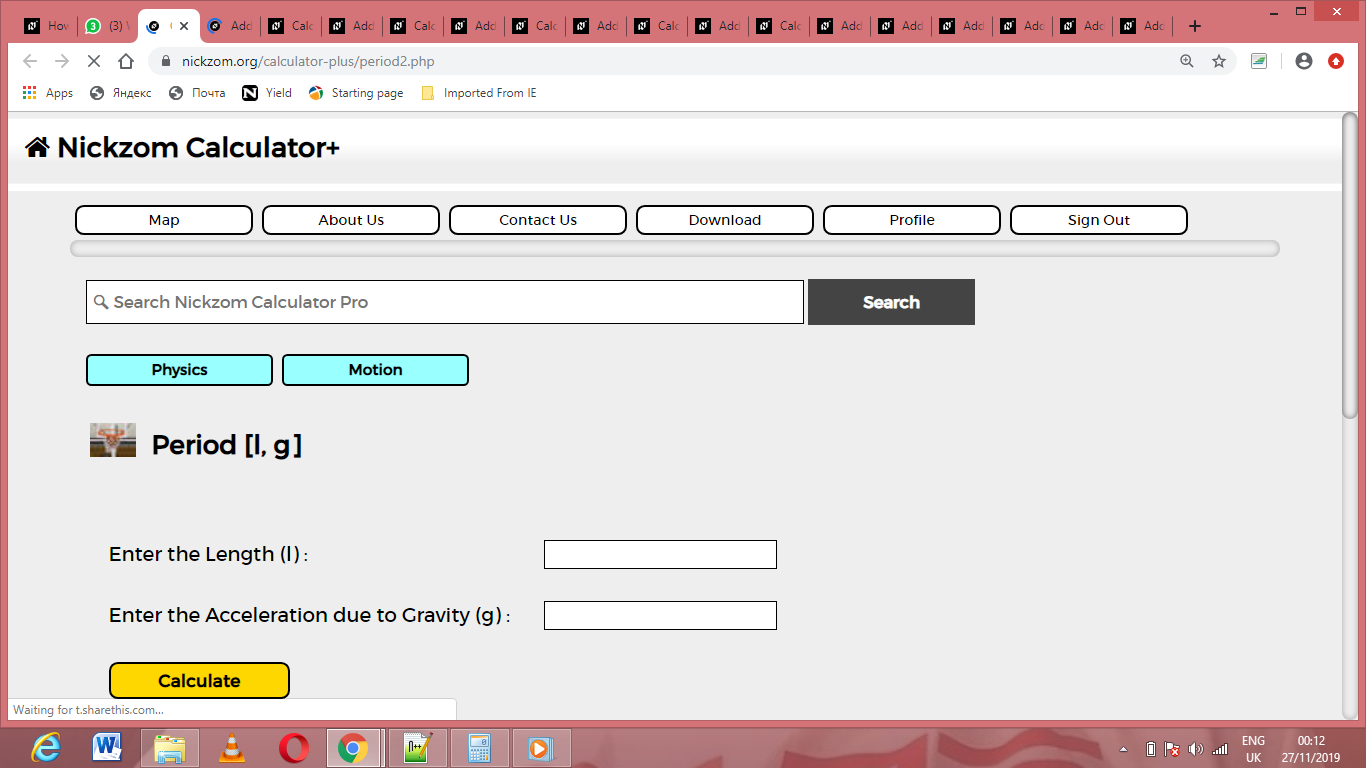Now, enter the values appropriately and accordingly for the parameters as required by the length (l) is and acceleration due to gravity (g) is 9.8.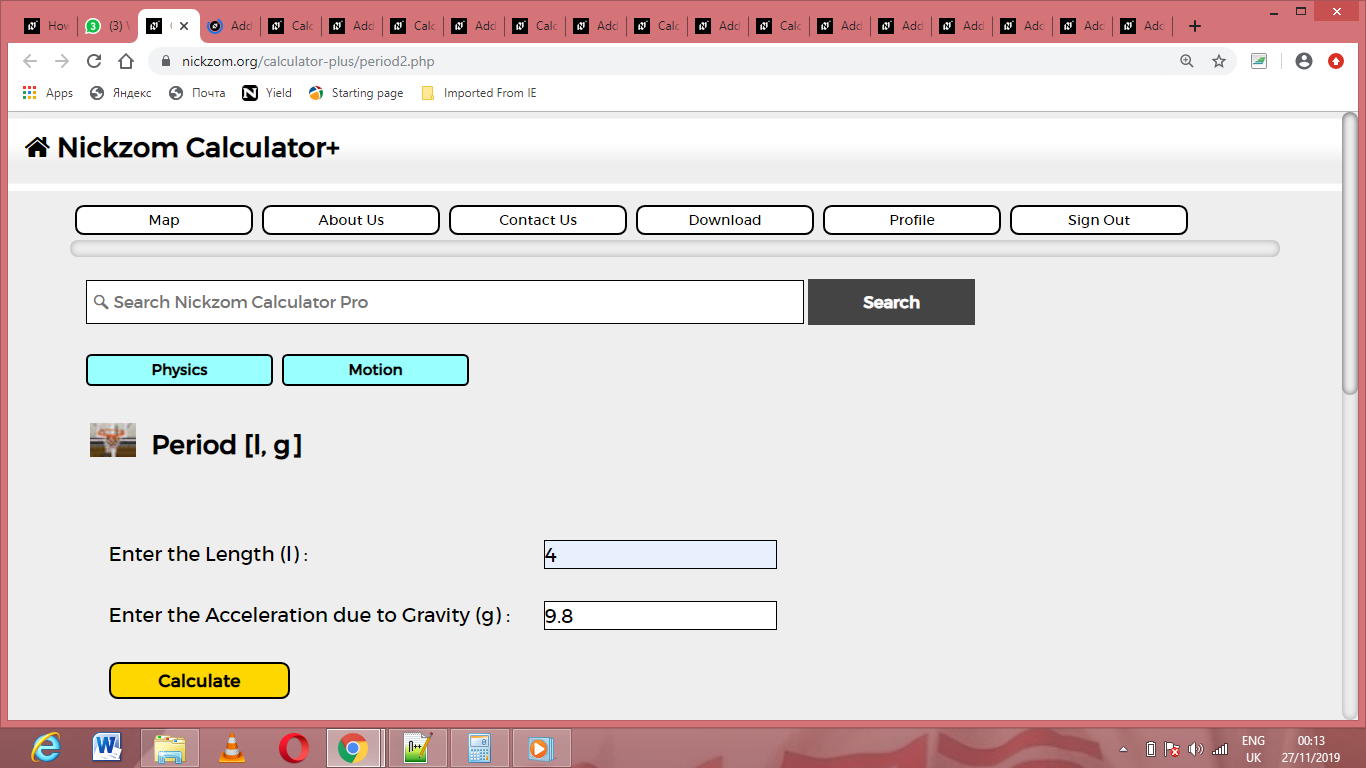Finally, Click on Calculate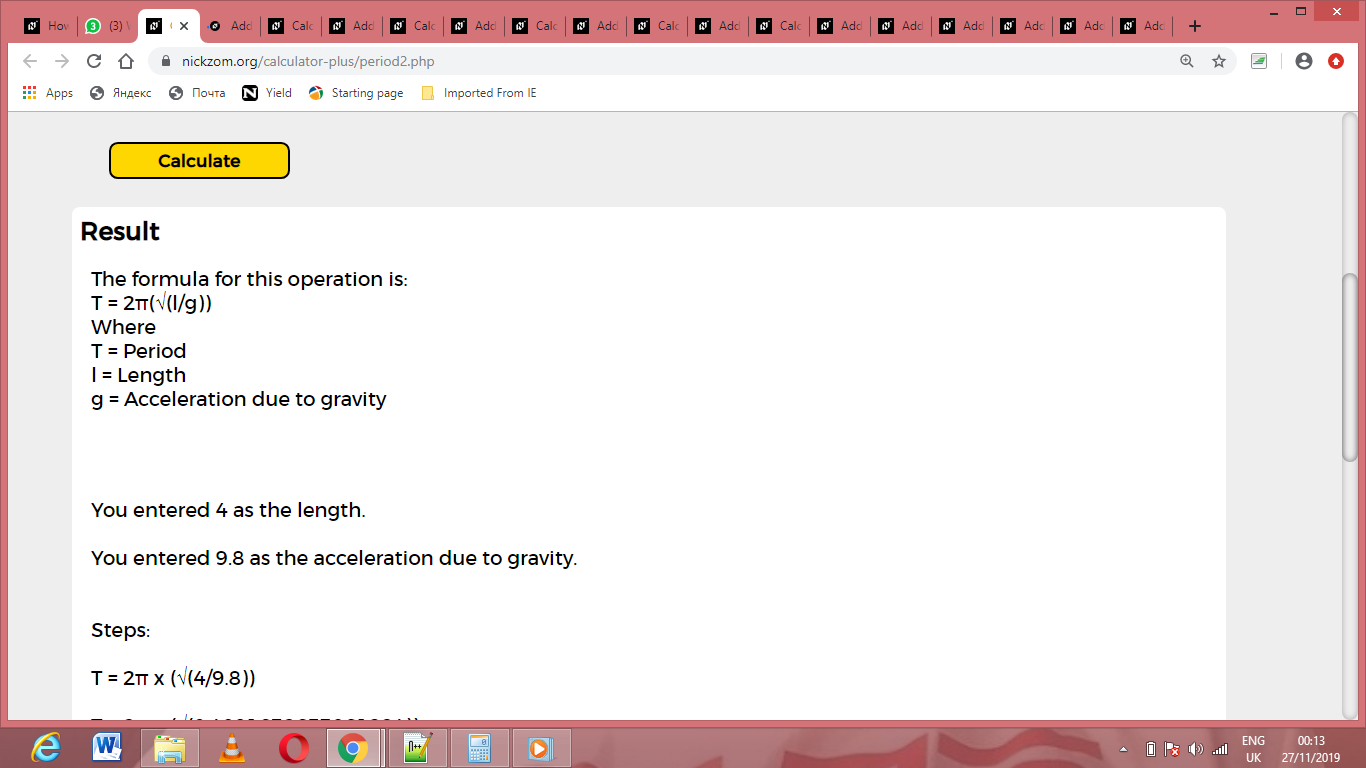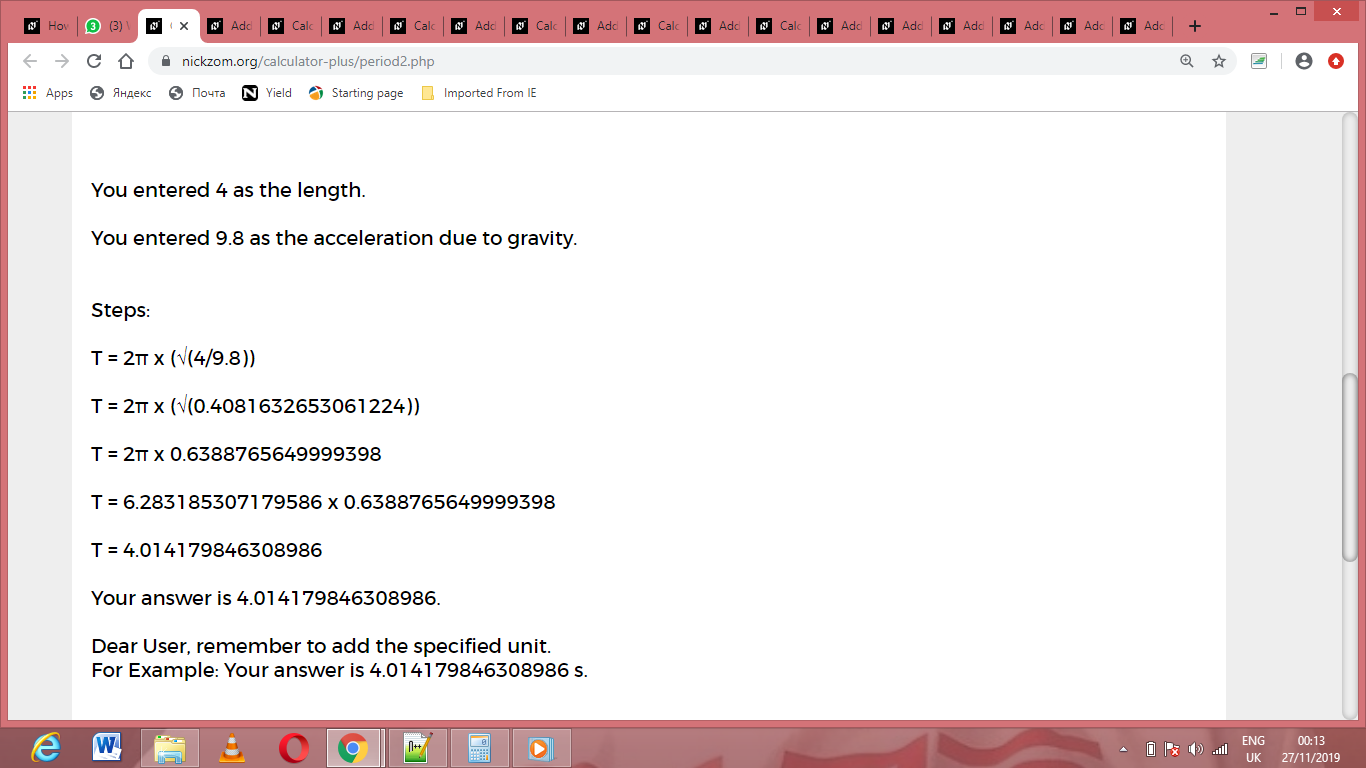As you can see from the screenshot above, Nickzom Calculator– The Calculator Encyclopedia solves for the period and presents the formula, workings and steps too.